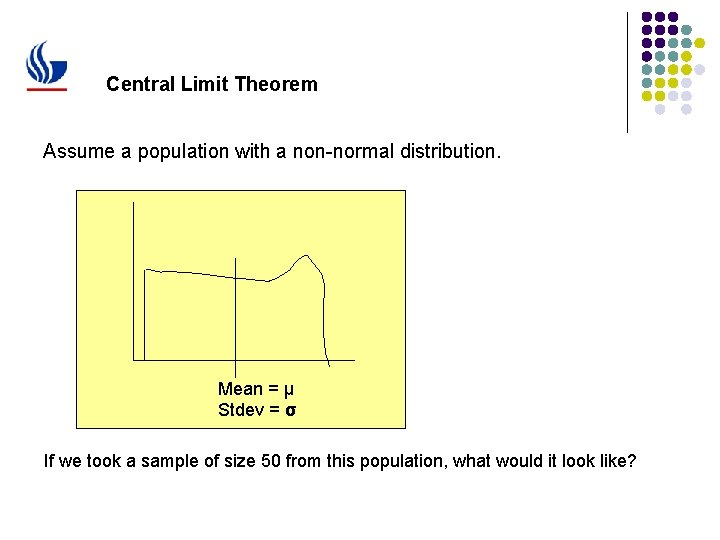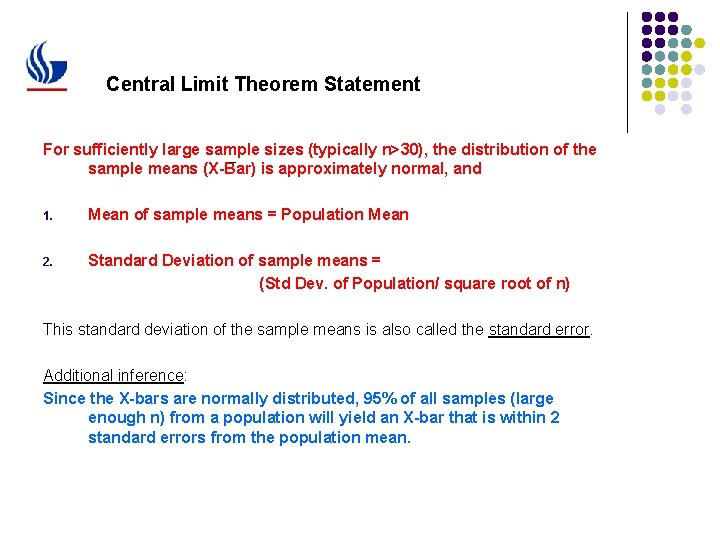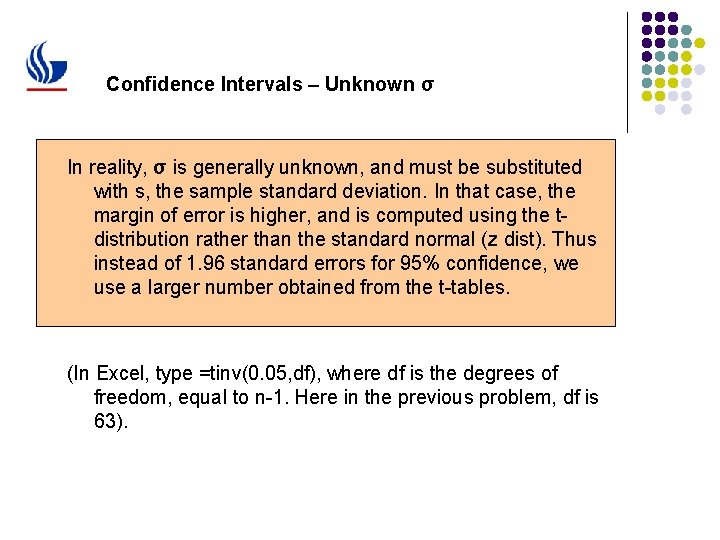# LSSG Black Belt Training Estimation Central Limit Theorem

• Slides: 7LSSG Black Belt Training Estimation: Central Limit Theorem and Confidence IntervalsCentral Limit Theorem Assume a population with a non-normal distribution. Mean = µ Stdev = σ If we took a sample of size 50 from this population, what would it look like?CLT - Multiple Samples from the same Population Each sample of n=50 from the same population will tend to look like the population, and the sample means will be close to the population mean. X 1 X 2 X 3 The sample means are unbiased estimators of the population mean. They will vary randomly above and below the actual population mean. If all such samples (n=50) were drawn, how would the sample means be distributed?CLT – Sampling Distribution of X Most sample means will be close to the population mean. l Some sample means will be a little farther away. l A few will be quite a bit off the mark. l A rare number will be extremely far away. In other words, the X values will be approximately normally distributed. l µx How would the mean of this distribution compare to the original population mean? How about the standard deviation of this distribution? How would sample size affect this relationship?Central Limit Theorem Statement For sufficiently large sample sizes (typically n>30), the distribution of the sample means (X-Bar) is approximately normal, and 1. Mean of sample means = Population Mean 2. Standard Deviation of sample means = (Std Dev. of Population/ square root of n) This standard deviation of the sample means is also called the standard error. Additional inference: Since the X-bars are normally distributed, 95% of all samples (large enough n) from a population will yield an X-bar that is within 2 standard errors from the population mean.Confidence Intervals We take a sample of 64 parts from a population, and want to estimate the population mean of the part length. The sample mean is 25 mm. The population standard deviation is known to be 0. 2 mm. From CLT, we know that this sample mean (25) is within 2 standard errors (actually 1. 96) of the population mean, with 95% confidence. Hence the reverse is also true. Thus, population mean is X-bar ± 2 * SE Here, SE = 0. 16 / √ 64 = 0. 16/8 = 0. 02 Thus 95% CI for µ is given by 25 ± 2*0. 02, or 25 ± 0. 04 mm The value 0. 04 is the Margin of Error (MOE)Confidence Intervals – Unknown σ In reality, σ is generally unknown, and must be substituted with s, the sample standard deviation. In that case, the margin of error is higher, and is computed using the tdistribution rather than the standard normal (z dist). Thus instead of 1. 96 standard errors for 95% confidence, we use a larger number obtained from the t-tables. (In Excel, type =tinv(0. 05, df), where df is the degrees of freedom, equal to n-1. Here in the previous problem, df is 63).